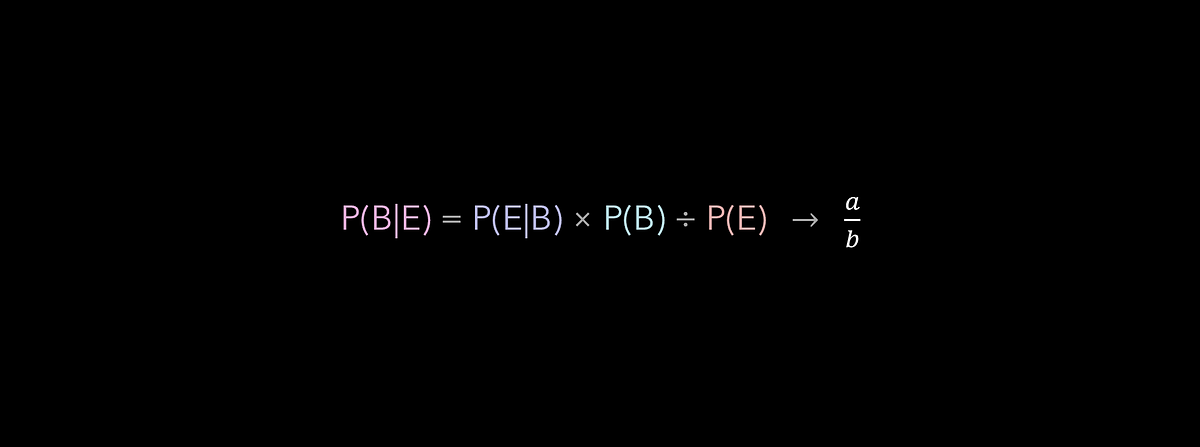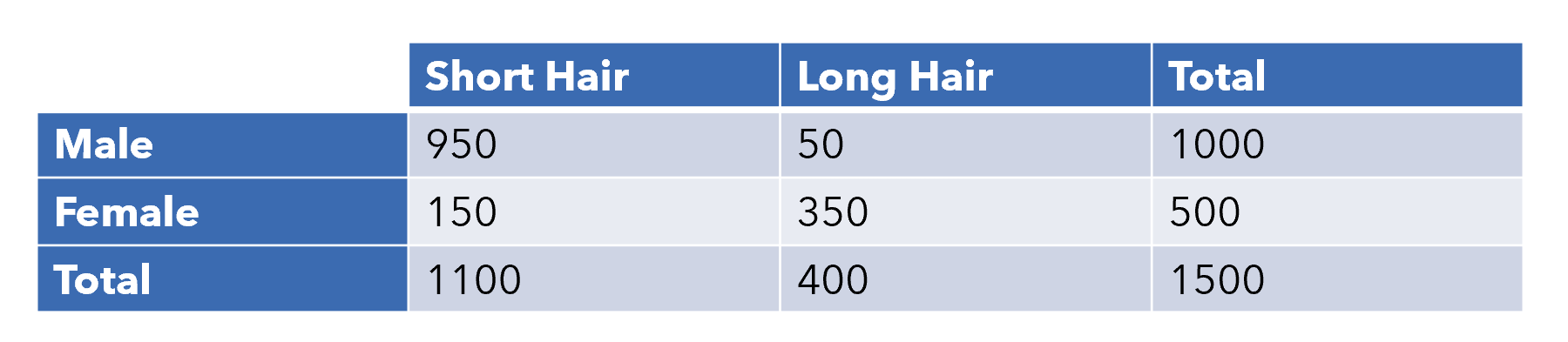# Bayes’ Theorem is Actually an Intuitive FractionPicking the Theorem apart, without the jargon. Bayes’ Theorem is one of the most known to the field of probability, and it is used often as a baseline model in machine learning. It is, however, too often memorized and chanted by people who don’t really know what P(B|E) = P(E|B) * P(B) / P(E) actually does.

Bayes’ Theorem is one of the most known to the field of probability, and it is used often as a baseline model in machine learning. It is, however, too often memorized and chanted by people who don’t really know what `P(B|E) = P(E|B) * P(B) / P(E)` actually does. This short article will pick apart Bayes’ Theorem and show how it simplifies to an intuitive fraction we all use on a common basis.

First, some basic probability context.

The belief is a statement we would like to verify is correct or incorrect, like ‘a person is male’ or ‘a person has long hair’. The evidence is known information about the subject in the belief. Lastly, the `|` vertical pipe is used as the word ‘given…’. You’ll often see `(B|E)` as ‘the probability belief B is true, given the evidence E.’

Let’s take the following table of students at Hypothetical High School.We want to find the probability that a person is female (belief) given long hair (evidence). This can be expressed as `(female|long hair)`. Even without the theorem, this is quite simple to calculate, intuitively. We simply need to divide the number of females who have long hair by the number of males who have long hair, which is `350/400=0.875`.

It’s worthwhile diving a little into why we have this intuition. Since we know that the person has long hair, we look in the column of ‘Long Hair’ and the two classes within that category (being male or female). Then, our formula is simply people who are female and who have long hair divided by all people who have long hair.

Hence, we can confidently say that, if you have long hair, you have a 87.5% chance of being a female at Hypothetical High School.

Let’s use Bayes’ Theorem to solve this — and you’ll realize that it is simply putting into rigorously mathematical terms this intuition!

Variable names from `a`-`i` will be used to represent different quantities. Remember that our intuitive formula was `e/h`, or the number of people who are female and have long hair, divided by the total number of people with long hair.

## 15 Machine Learning and Data Science Project Ideas with Datasets

Learning is a new fun in the field of Machine Learning and Data Science. In this article, we’ll be discussing 15 machine learning and data science projects.

## Applied Data Analysis in Python Machine Learning and Data Science | Scikit-Learn

Applied Data Analysis in Python Machine learning and Data science, we will investigate the use of scikit-learn for machine learning to discover things about whatever data may come across your desk.

## Difference between Machine Learning, Data Science, AI, Deep Learning, and Statistics

In this article, I clarify the various roles of the data scientist, and how data science compares and overlaps with related fields such as machine learning, deep learning, AI, statistics, IoT, operations research, and applied mathematics.

## Most popular Data Science and Machine Learning courses — July 2020

Most popular Data Science and Machine Learning courses — August 2020. This list was last updated in August 2020 — and will be updated regularly so as to keep it relevant

## Statistics for Data Science

Statistics for Data Science and Machine Learning Engineer. I’ll try to teach you just enough to be dangerous, and pique your interest just enough that you’ll go off and learn more.